# R Weekly Bulletin Vol – XIVThis week’s R bulletin covers some interesting ways to list functions, to list files and illustrates the use of double colon operator. Hope you like this R weekly bulletin. Enjoy reading!

### Shortcut Keys

1. New document - Ctrl+Shift+N
2. Close active document - Ctrl+W
3. Close all open documents - Ctrl+Shift+W

### Problem Solving Ideas

#### How to list functions from an R package

We can view the functions from a particular R package by using the “jwutil”s package. Install the package and use the lsf function from the package. The syntax of the function is given as:

lsf(pkg)

Where pkg is a character string containing package name.

The function returns a character vector of function names in the given package.

Example:

library(jwutil)
library(rowr)
lsf("rowr")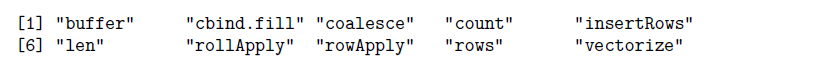#### How to list files with a particular extension

To list files with a particular extension, one can use the pattern argument in the list.files function. For example to list CSV files use the following syntax:

Example:

# This will list all the csv files present in the current working directory.
# To list files in any other folder, you need to provide the folder path.

files = list.files(pattern = "\\.csv$")#$ at the end means that this is end of the string.
# Adding \. ensures that you match only files with extension .csv

list.files(path = "C:/Users/MyFolder", pattern = "\\.csv\$")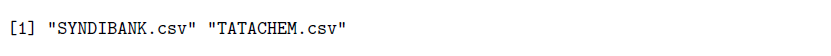#### Using the double colon operator

The double colon operator is used to access exported variables in a namespace. The syntax is given as:

pkg::name

Where pkg is the package name symbol or literal character string. The name argument is the variable name symbol or literal character string.

The expression pkg::name returns the value of the exported variable from the package if it has a namespace. The package will be loaded if it was not loaded already before the call. Using the double colon operator has its advantage when we have functions of the same name but from different packages. In such a case, the sequence in which the libraries are loaded is important.

To see the help documentation for these colon operators you can run the following command in R - ?'::' or help(":::")

Example:

library("dplyr")

first = c(1:6)
second = c(3:9)

dplyr::intersect(first, second)
 3 4 5 6
base::intersect(first, second)
 3 4 5 6

In this example, we have two functions having the same names but from different R packages. In some cases, functions having same names can produce different results. By specifying the respective package name using the double colon operator, R knows in which package to look for the function.

### Functions Demystified

#### path.package function

The path.package function returns path to the locations where the given package is found. If the package is not mentioned then the function will return the path of the all the currently attached packages. The syntax of the function is given as:

path.package(package, quiet = FALSE)

The quiet argument takes a default value of False. If this is changed to True then it will throw a warning if the package named in the argument is not attached and will give an error if none are attached.

Example:

path.package("stats")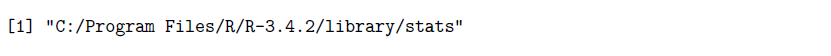#### fill.na function

There are different R packages which have functions to fill NA values. The fill.na function is part of the mefa package and it replaces NA values with the nearest values above them in the same column.The syntax of the function is given as:

fill.na(x)

Where, x can be a vector, a matrix or a data frame.

Example:

library(mefa)
x = c(12,NA,15,17,21,NA)
fill.na(x)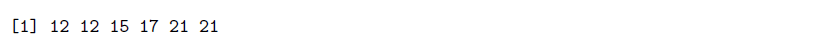#### rank function

The rank function returns the sample ranks of the values in a vector. Ties (i.e., equal values) and missing values can be handled in several ways.

rank(x, na.last = TRUE, ties.method = c("average", "first", "random", "max","min"))

where, x: numeric, complex, character or logical vector na.last: for controlling the treatment of NAs. If TRUE, missing values in the data are put last; if FALSE, they are put first; if NA, they are removed; if “keep” they are kept with rank NA ties.method: a character string specifying how ties are treated

Examples:

x = c(3, 5, 1, -4, NA, Inf, 90, 43)
rank(x)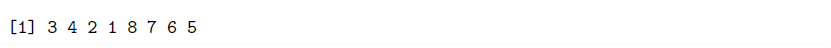rank(x, na.last = FALSE)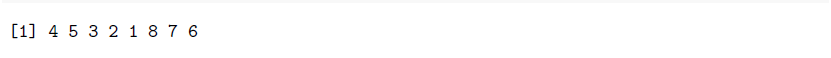### Next Step

A Step-by-step tutorial to use RExcel for implementing functions of R in Excel. Learn how to transfer data seamlessly between R and Excel using RExcel. Click here to read now.